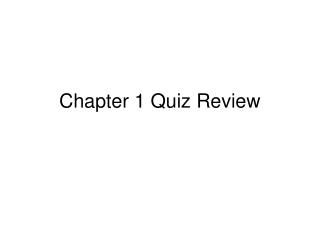Download PresentationChapter 1 Quiz Review

Chapter 1 Quiz Review - PowerPoint PPT Presentation

Chapter 1 Quiz Review. Name a line that contains point F. Give another name for line n. Name three collinear points. Name the plane containing lines n and p. Find the measure of the given segment. (draw a picture, show all work, and include labels) Point H is between points G and I.I am the owner, or an agent authorized to act on behalf of the owner, of the copyrighted work described.
Download PresentationChapter 1 Quiz Review

Download Policy: Content on the Website is provided to you AS IS for your information and personal use and may not be sold / licensed / shared on other websites without getting consent from its author.While downloading, if for some reason you are not able to download a presentation, the publisher may have deleted the file from their server.

- - - - - - - - - - - - - - - - - - - - - - - - - - E N D - - - - - - - - - - - - - - - - - - - - - - - - - -
Presentation Transcript
1. Chapter 1 Quiz Review

2. Name a line that contains point F. Give another name for line n. Name three collinear points. Name the plane containing lines n and p.

3. Find the measure of the given segment. (draw a picture, show all work, and include labels) • Point H is between points G and I. GH = 12.5 in GI = 17.3 in HI = ?

4. Find the value of the variable and XY, if Z is between X and Y. (draw a picture then solve) XZ = 5b + 1 ZY = 4b – 5 XZ = 11

5. In the figure, ray GK bisects angle FGH • Find y if angle FGK = 6a +7 and angle KGH = 8a - 2

6. In the figure rays JK and KL are opposite rays, angles 1 and 2 are congurent and ray KM bisects angle NKL What bisects angle JKN? If angle JKM = 5x + 8 and angle 4 = x, what is the measure of angle 4? If angle JKN is a right angle and angle 1 = 4t + 5, what is t?

7. Answers • Name a line that contains point F. • FG, GF, line p • Give another name for line n. • AD, AC, CA, CD, DC, DA • Name three collinear points. • A, C & D or J, D, & B • Name the plane containing lines n and p. • Plane K

8. Answers • Find the measure of the given segment. (draw a picture, show all work, and include labels) • Point H is between points G and I. GH = 12.5 in GI = 17.3 in HI = ? G 12.5 in x I H 17.3 in 12.5 + x = 17.3 -12.5 -12.5 x = 4.8 HI = 4.8 in

9. Answers • Find the value of the variable and XY, if Z is between X and Y. (draw a picture then solve) XZ = 5b + 1 ZY = 4b – 5 XZ = 11 x 5b + 1 4b - 5 Y Z 11 XY = 5(2) + 1 + 4(2) – 5 = 10 + 1 + 8 – 5 XY = 14 5b + 1 = 11 - 1 - 1 5b = 10 /5 /5 b = 2

10. Answers • Find the distance and midpoint for the given segment. KN Distance: KN = 3 - -3 = 6 Midpoint: 3 + -3 2 = 0

11. Answers • Find the distance and midpoint for the segment between A(-3, 7) and B(6, 2)

12. Answers • In the figure, ray GK bisects angle FGH • Find y if angle FGK = 6a +7 and angle KGH = 8a - 2 6a + 7 6a + 7 = 8a – 2 -6a -6a 7 = 2a – 2 + 2 + 2 9 = 2a /2 /2 a = 4.5 8a - 2 y = 8(4.5) – 2 = 36 – 2 = 34o

13. Answers • In the figure rays JK and KL are opposite rays, angles 1 and 2 are congurent and ray KM bisects angle NKL What bisects angle JKN? KP If angle JKM = 5x + 8 and angle 4 = x, what is the measure of angle 4? If angle JKN is a right angle and angle 1 = 4t + 5, what is t? 5x + 8 + x = 180 6x + 8 = 180 - 8 -8 6x = 172 /6 /6 x = 28.666…. 2(4t + 5) = 90 8t + 10 = 90 8t = 80 t = 8